##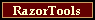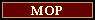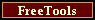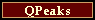What is the difference between the Sciex and the Micromass Maximum Entropy algorithms?  And which Maximum Entropy algorithm is used by MOP?

# Maximum Entropy for ESI Mass Spectrometry - Summary

Both the Sciex and the Micromass Maximum Entropy algorithms for deconvolution of electrospray mass spectrometry data answer the question: What is the most probable parent mass spectrum, given the data in front of me?  Both use the same knowledge of the spectrometer: it creates multiple images and it smears the data.  The only differences between the algorithms are the assumptions about the noise.  The Micromass Maxent algorithm assumes Normal (gaussian) noise statistics.  The Sciex BioSpect Reconstruct algorithm assumes Poisson (counting) noise statistics.   The principles and equations for both algorithms are given.  MOP also performs Maximum Entropy deconvolution for Poisson (counting) data.  MOP uses the same RazorMOP engine as Sciex.

# The Most Probable Parent Mass Spectrum

The data from a mass spectrometer produce an apparent mass spectrum y(m/z), where y is the number of counts at apparent mass m/z. The data have noise.  There will be electronic noise from the detector and Poisson noise from the counting statistics.

A good spectroscopist will ask the appropriate question:

What is the most probable parent mass spectrum o, given this data y?

Mathematically, maximize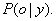The solution requires Bayes Rule,as well as an informed a priori probability for the parent mass spectrum## A Prior Probability for the Parent Mass Spectrum

The multinomial probability law is used as the prior probability for the parent mass spectrum,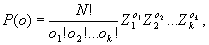whereis the user's initial estimate of the parent mass spectrum. (Usually, one choses a flat prior,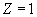, for the initial estimate of the parent mass distribution).

## Probability of the Data

The data y(m/z) are related to the parent mass spectrum o, and the noise n, by an equation that describes both the characteristics of the spectrometer and the statistics of the noise.  The equation for noiseless data a(m/z) isThe transform Q describes all the properties and limitations of the spectrometer.  Q includes multiple-imaging, for electrospray ionization, and it includes any smearing of the mass spectrum due to focussing or instrument imperfections.  Q may also include the isotopic pattern or isotopic width of the parent mass, if resolution enhancement of parent masses is required.

## Probability of the Data for Normal (gaussian) noise

The probability for a data set y, given a parent mass spectrum o, when the noise is described by Normal statistics is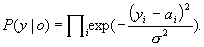The Micromass algorithm uses this Normal-noise equation.

## Probability of the Data for Poisson (counting) noise

The probability for a data set y, given a parent mass spectrum o, when the noise is described by Poisson statistics is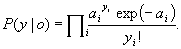The Sciex algorithm uses this Poisson-noise equation.

# The Final Maximum Entropy Equations

The mathematical problem, which has been stated in this way,

Maximize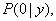can also be solved by maximizing the logarithm of the probability, as such:

Maximize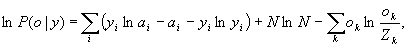for Poisson statistics.

Maximizefor Normal statistics.

Both of these equations are Maximum Entropy equations, because both contain the entropy term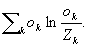Both equations provide the most probable parent mass distribution, one for the case of Normal noise statistics, the other for the case of Poisson statistics.